# Introduction to theft

#### 2023-03-27

library(theft)

## Purpose

theft facilitates user-friendly access to a structured analytical workflow for the extraction, analysis, and visualisation of time-series features. This structured workflow is presented in the graphic below (note that theft has many more functions than displayed in this graphic — keep reading for more):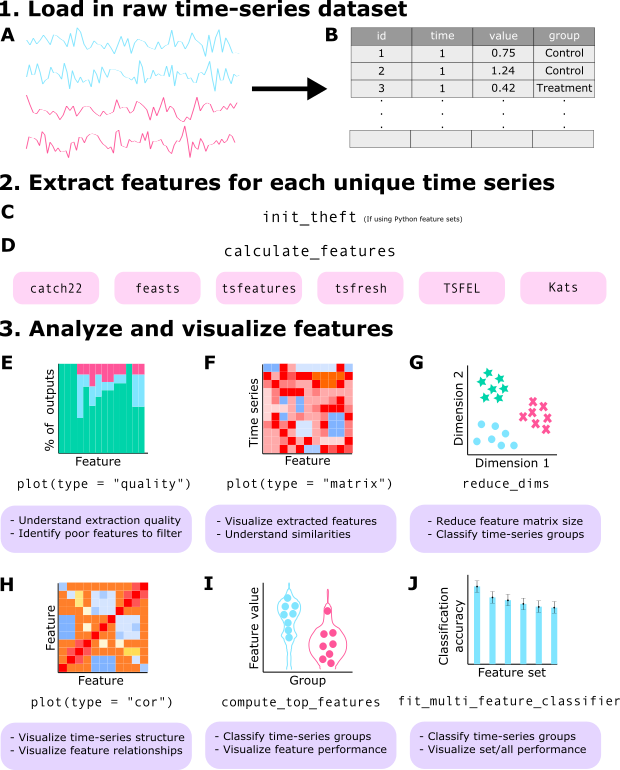## Core calculation functions

To explore package functionality, we are going to use a dataset that comes standard with theft called simData. This dataset contains a collection of randomly generated time series for six different types of processes. The dataset can be accessed via:

theft::simData

The data follows the following structure:

head(simData)
#>                      values timepoint               id        process
#> Gaussian Noise.1 -0.6264538         1 Gaussian Noise_1 Gaussian Noise
#> Gaussian Noise.2  0.1836433         2 Gaussian Noise_1 Gaussian Noise
#> Gaussian Noise.3 -0.8356286         3 Gaussian Noise_1 Gaussian Noise
#> Gaussian Noise.4  1.5952808         4 Gaussian Noise_1 Gaussian Noise
#> Gaussian Noise.5  0.3295078         5 Gaussian Noise_1 Gaussian Noise
#> Gaussian Noise.6 -0.8204684         6 Gaussian Noise_1 Gaussian Noise

### Calculating feature summary statistics

The core function that automates the calculation of the feature statistics at once is calculate_features. You can choose which subset of features to calculate with the feature_set argument. The choices are currently "catch22", "feasts", "Kats", "tsfeatures", "tsfresh", and/or "TSFEL".

Note that Kats, tsfresh and TSFEL are Python packages. The R package reticulate is used to call Python code that uses these packages and applies it within the broader tidy data philosophy embodied by theft. At present, depending on the input time-series, theft provides access to $$>1200$$ features. Prior to using theft (only if you want to use the Kats, tsfresh or TSFEL feature sets - the R-based sets will run fine) you should have a working Python installation and download Kats using the instructions located here, tsfresh here or TSFEL here.

Here is an example with the catch22 set:

feature_matrix <- calculate_features(data = simData,
id_var = "id",
time_var = "timepoint",
values_var = "values",
group_var = "process",
feature_set = "catch22",
seed = 123)

Note that for the catch22 set you can set the additional catch24 argument to calculate the mean and standard deviation in addition to the standard 22 features:

feature_matrix <- calculate_features(data = simData,
id_var = "id",
time_var = "timepoint",
values_var = "values",
group_var = "process",
feature_set = "catch22",
catch24 = TRUE,
seed = 123)

Note that if you want to use any of the Python-based packages, you must first tell R (prior to running calculate_features) which Python and/or virtual environment on your computer contains the installed libraries. This can be done in theft via the init_theft function, which has two arguments:

1. python_path — string specifying the filepath to the version of Python you wish to use
2. venv_path — string specifying the filepath to the Python virtual environment where tsfresh, TSFEL, and/or Kats are installed

However, you do not necessarily have to use this convenience function. If you have another method for pointing R to the correct Python (such as reticulate or findpython), you can use those in your workflow instead.

NOTE: You only need to call init_theft or your other solution once per session.

A tidy dataframe of most of the included features and the set they correspond to is available in the dataframe feature_list:

head(feature_list)
#>   feature_set                  feature
#> 1     catch22       DN_HistogramMode_5
#> 2     catch22      DN_HistogramMode_10
#> 3     catch22                CO_f1ecac
#> 4     catch22           CO_FirstMin_ac
#> 5     catch22 CO_HistogramAMI_even_2_5
#> 6     catch22            CO_trev_1_num

NOTE: If using the tsfresh feature set, you might want to consider the tsfresh_cleanup argument to calculate_features. This argument defaults to FALSE and specifies whether to use the in-built tsfresh relevant feature filter or not.

For a detailed comparison of the six feature sets, see this paper for a detailed review1.

## Data quality checks

The calculate_features function returns an object of class feature_calculations. Objects of this type are purposefully looked-for by other functions in theft. Because it is a class, simple methods such as plot() can be called on the object to produce a range of statistical graphics. The first is a visualisation of the data types of the calculated feature vectors. This is useful for inspecting which features might need to be dropped due to large proportions of undesirable (e.g., NA, NaN etc.) values. We can specify the plot type = "quality to make this graphic:

plot(feature_matrix, type = "quality")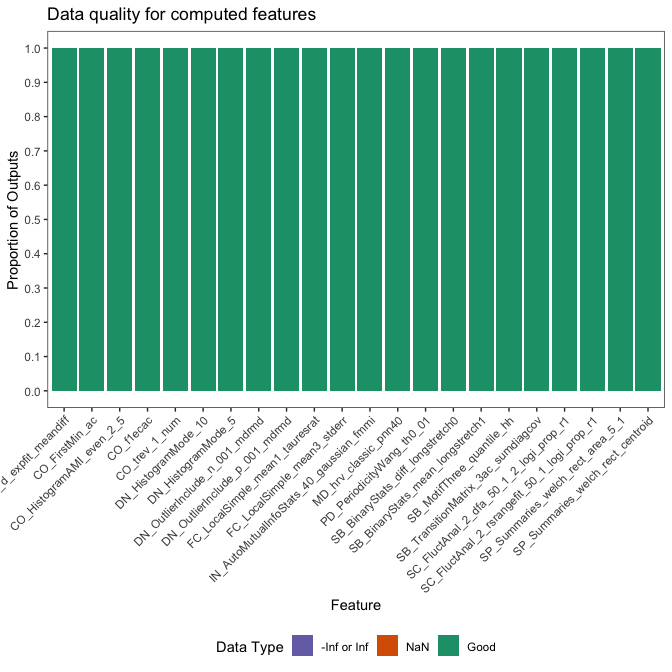## Normalising/scaling functions

Putting calculated feature vectors on an equal scale is crucial for any statistical or machine learning model as variables with high variance can adversely impact the model’s capacity to fit the data appropriately, learn appropriate weight values, or minimise a loss function. theft includes function normalise to rescale either the whole feature_calculations object, or a single vector of values (e.g. values for all participants on just the SB_BinaryStats_mean_longstretch1 feature). Four normalisation methods are offered:

• z-score - "z-score"
• Sigmoid - "Sigmoid"
• Outlier-robust Sigmoid (credit to Ben Fulcher for creating the original MATLAB version) - "RobustSigmoid"
• Min-max - "MinMax"

Normalisation on the whole feature_calculations object can be performed in one line:

normed <- normalise(feature_matrix, method = "z-score")

For single vector normalisation, all you need to do is pass in a vector as normalise checks for object classes.

## Data visualisation and low-dimensional project functions

The package also comes with additional statistical and graphical functionality:

• Feature by time-series matrix as a heatmap
• Low dimensional projections of the feature space and plotting as a scatterplot
• Pairwise feature correlation matrix as a heatmap

### Feature matrices

The function calling type = "matrix" in plot() on a feature_calculations object takes itand produces a ggplot object heatmap showing the feature vectors across the x axis and each time series down the y axis. Prior to plotting, the function hierarchically clusters the data across both rows and columns to visually highlight the empirical structure. Note that you have several options for the hierarchical clustering linkage algorithm to use:

• "average" (default)
• "ward.D"
• "ward.D2"
• "single"
• "complete"
• "mcquitty"
• "median"
• "centroid"

See the hclust documentation for more information.

Note that the legend for this plot (and other matrix visualisations in theft) have been discretised for visual clarity as continuous legends can be difficult to interpret meaningful value differences easily.

plot(feature_matrix, type = "matrix")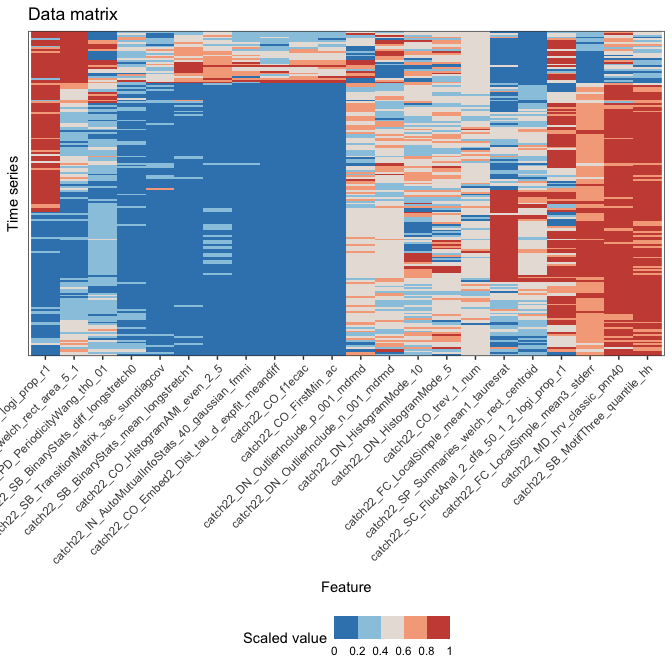You can control the normalisation type with the method argument and the hierarchical clustering method with the clust_method argument (the example above used defaults so manual specification was not needed).

### Low dimensional projections

The function reduce_dims takes the feature_calculations object and calculates either a principal components analysis (PCA) or t-distributed stochastic neighbour embedding (t-SNE) fit on it. This result is stored in a custom object class called low_dimension:

low_dim <- reduce_dims(feature_matrix,
method = "RobustSigmoid",
low_dim_method = "PCA",
seed = 123)

We can similarly call plot() on this object to produce a two-dimensional scatterplot of the results:

plot(low_dim)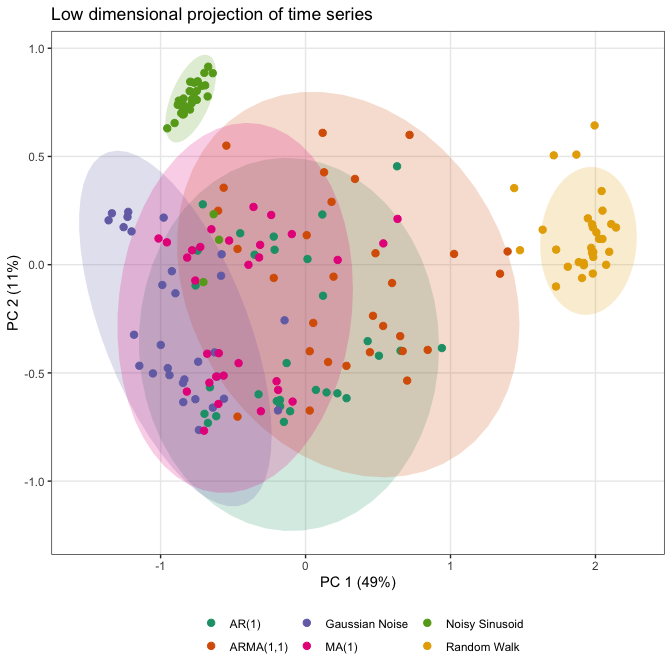Alternatively, a t-SNE version can be specified in a similar fashion, with the perplexity hyperparameter able to be controlled by the user. Typical values for this range between 5 and 50, depending on the size of the data. At lower levels of perplexity, local variations tend to dominate, while at very high levels of perplexity, results can be uninterpretable as clusters can merge. See this interactive article for a detailed review. Shaded covariance ellipses can also be disabled when plotting low_dimension objects by setting show_covariance = FALSE:

low_dim2 <- reduce_dims(feature_matrix,
method = "RobustSigmoid",
low_dim_method = "t-SNE",
perplexity = 10,
seed = 123)

plot(low_dim2, show_covariance = FALSE)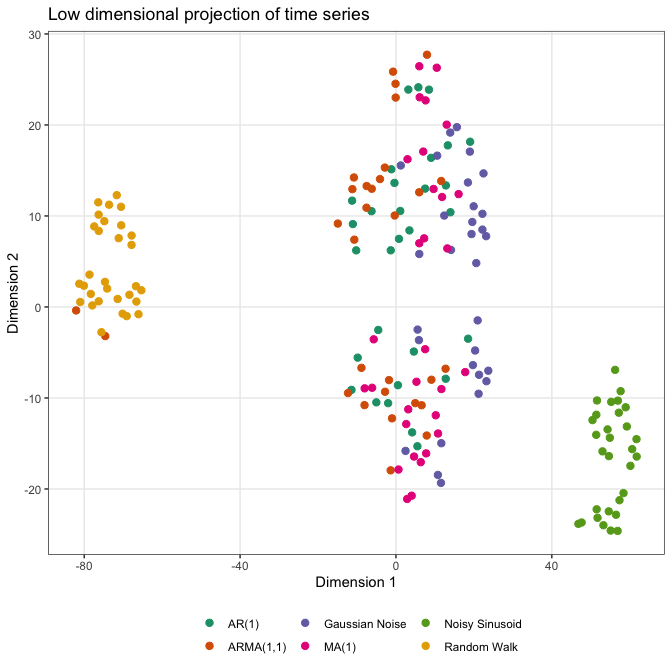### Pairwise correlations

You can plot correlations between feature vectors using plot(type = "cor") on a feature_calculations object:

plot(feature_matrix, type = "cor")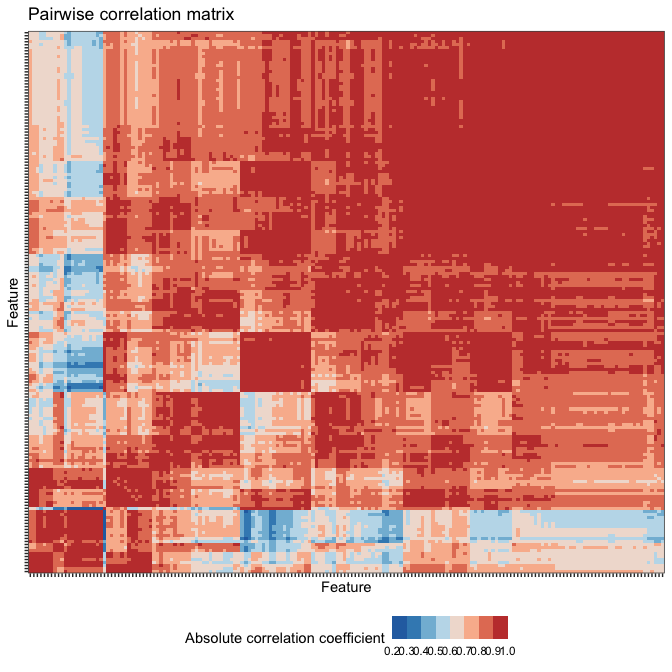Similarly, you can control the normalisation type with the method argument and the hierarchical clustering method with the clust_method argument (the example above used defaults so manual specification was not needed).

## Time-series classification

### Feature-by-feature

Since feature-based time-series analysis has shown particular promise for classification problems, theft includes functionality for exploring group separation in addition to the low dimensional representation. The compute_top_features function takes a feature_calculations object and performs the following:

1. Processes the data ready for analysis
2. Computes a classification algorithm or statistical model on the entire input feature_calculations object
3. Extracts individual feature performance from the classification algorithm using a designated performance metric
4. Filters the feature data to the top n performing features (where n is specified by the user)
5. Plots a feature $$\times$$ feature correlation matrix as a heatmap for the top performing features
6. Plots a group of violin plots coloured by class label for the top performing features

This analysis is useful because it can help guide researchers toward more efficient and appropriate interpretation of high-performing features (if any exist) and helps protect against over-interpretation of feature values.

This function returns an object with three components to summarise the above steps:

1. ResultsTable – a dataframe containing feature names, feature set membership, and performance statistics for the top performing features
2. FeatureFeatureCorrelationPlot – a ggplot containing the pairwise correlations between top performing features represented as a heatmap
3. ViolinPlots – a ggplot containing a matrix of violin plots showing class discrimination by feature

The code below produces analysis for a multiclass problem using a Gaussian process classifier with a radial basis function kernel. It implements a custom “empirical null” procedure for estimating a p-value based on classification accuracy of the real data compared to a distribution of null samples built from classification accuracies of the same data but with random class label shuffles. This is also known as permutation testing (see this document2 for a good overview). Note that higher numbers of permutation samples means that a better empirical null distribution can be generated. It is recommended to run 100-1000 permutations, though this comes with considerable computation time. We’ll enable a k-fold cross-validation procedure too for good measure. Note that using these procedures increases the computation time. Electing to not use an empirical null will be faster, but top features will be determined based off classification accuracy of features and not p-values. Further, when use_k_fold = FALSE, the model will instead be fit on all data and predict classes based off the same data (in caret language, this is equivalent to caret::trainControl(method = "none") and then calling predict on the input data and getting accuracy from the confusion matrix between the two). This will very likely lead to overfitting, but will return results orders of magnitude faster than if use_k_fold = TRUE.

Importantly, the argument null_testing_method has two choices:

1. ModelFreeShuffles – Generates num_permutations number of random shuffles of group labels and computes the proportion that match, returning a distribution of “accuracies”. This model is extremely fast as it involves no null model fitting
2. NullModelFits – Generates num_permutations number of models that are trained and tested on data where the class label is shuffled. This method is slow but fits separate null models for each num_permutations shuffle

We we only use ModelFreeShuffles throughout this tutorial to reduce computation time.

p_value_method also has two choices:

1. empirical – calculates the proportion of null classification accuracies that are equal to or greater than the main model accuracy
2. gaussian – calculates mean and standard deviation of the null distribution to analytically calculate p-value for main model against. Initial package testing indicated that the null distributions (especially for increasing num_permutations) were approximately Gaussian, meaning this approach can be feasibly used

Note that ModelFreeShuffles is incompatible with pool_empirical_null = TRUE as the null distributions would be exactly the same. Further, if you set pool_empirical_null = TRUE this will compute statistical analysis for each feature against the entire pooled empirical null classification results of all features. Setting it to FALSE will compute a p-value for each feature against only its empirical null distribution.

IMPORTANT NOTE: theft currently does not label any results as “statistically significant”. If you intend to in your work, please adjust for multiple comparisons when considering numerous results against a threshold (e.g., $$\alpha = 0.05$$) and ensure any decisions you make are grounded in careful thought.

The seed argument allows you to specify a number to fix R’s random number generator for reproducible results. It defaults to 123 if nothing is provided.

outputs <- compute_top_features(feature_matrix,
num_features = 10,
normalise_violin_plots = FALSE,
method = "RobustSigmoid",
cor_method = "pearson",
test_method = "svmLinear",
clust_method = "average",
use_balanced_accuracy = FALSE,
use_k_fold = TRUE,
num_folds = 3,
use_empirical_null =  TRUE,
null_testing_method = "ModelFreeShuffles",
p_value_method = "gaussian",
num_permutations = 3,
pool_empirical_null = FALSE,
seed = 123)

Each component is named and can be accessed through regular use of the $ operator. Here is the feature-feature correlation plot: print(outputs$FeatureFeatureCorrelationPlot)